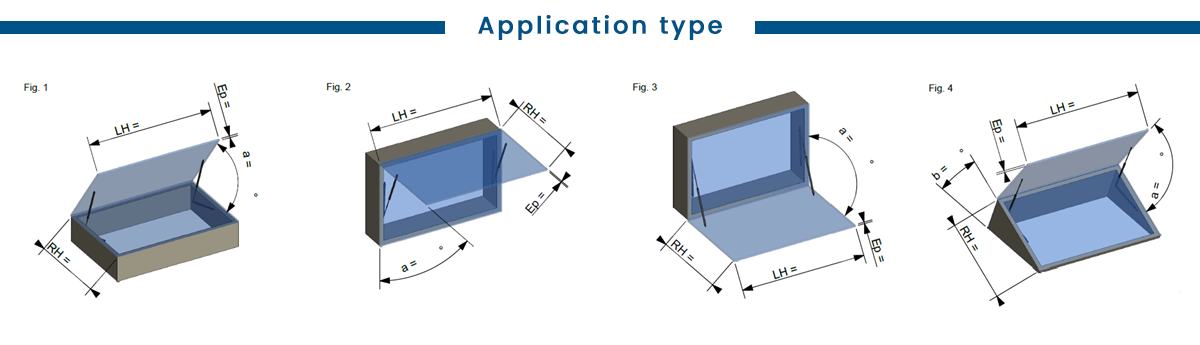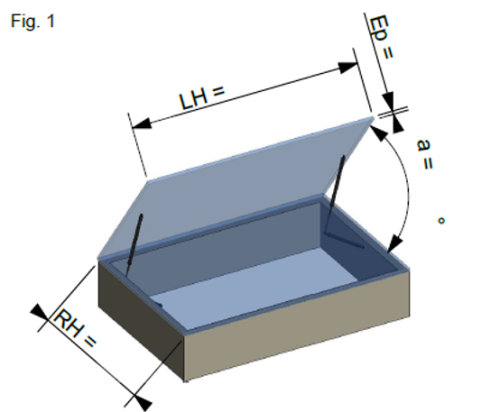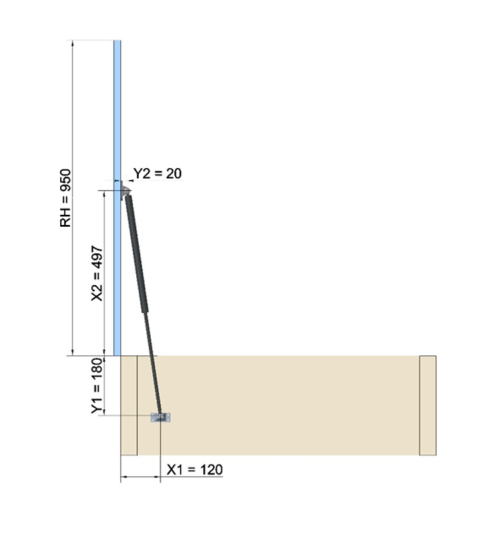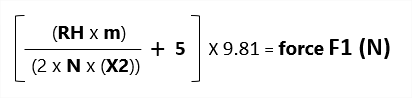Home > Installing a gas spring in an application

# Installing a gas spring in an application

Please note that the more information you have, the more accurate and therefore efficient the gas spring will be.

First of all, it is necessary to know the environment in which the gas springs will evolve : temperature, dust, aggressive environment, food etc..

The dimensions of the moving part (RH and LH) and the Weight to be lifted should be taken into account when choosing the diameter of the gas spring rod and the type of end fitting (environment, frequency of use, operator etc.).## Case study with Fig. 1

• Application type: Floor hatch – Ground level trapdoor (Most common)

• To open a door with a 90° opening angle, the following rule must be observed: Stroke = 1/3 of RH

Exemple

• RH = 950mm, LH = 1500mm, Weight = 30Kg, Opening angle = 90°, No of gas springs
• Stroke: 950:3= 316mm => see our catalogue to choose the closest stroke = in this case : 300mm

• We can use the reference: ST 300 +F1+ D10

Fixing

Important: All the dimensions are taken from the axis of rotation

•       On the frame: The dimension Y1 will be less than the stroke, X1 will give the gas spring the necessary angle to the lever arm to start the movement, an ideal position will give an angle of 15° to 25° to the gas spring when the door is closed.

On the opening

The dimension X2 will be defined by the extended length of the gas spring and the maximal opening angle.

Y2 will take into account the thickness of the door as well as the type of fixing bracket chosen.

In this example: X2 = 497mm, Y2 = 20mm

If all the parameters are accurate, the hatch should be closed with is own weight.*Les côtes sont exprimées en mm

## Force calculation

To calculate the force F1 of a gas spring in these two cases, the following formula should be applied:Adjust the force at upper tolerance:

F1 =     30 < N <     50      Add     +0N

F1 =     50 < N <   250      Add   +20N

F1 =   250 < N <   750     Add   +30N

F1 =   750 < N < 1500     Add   +60N

F1 = 1500 < N < 3000     Add +150N

F1 = 3000 < N < 6000     Add +300N